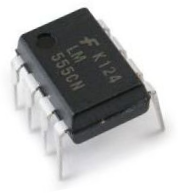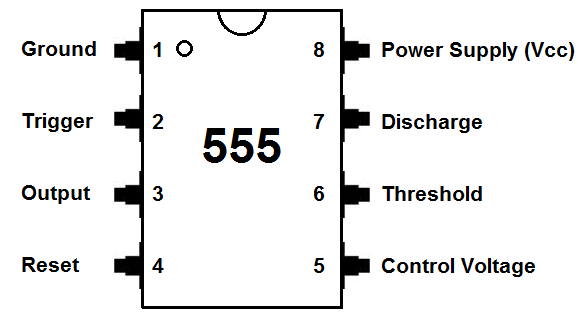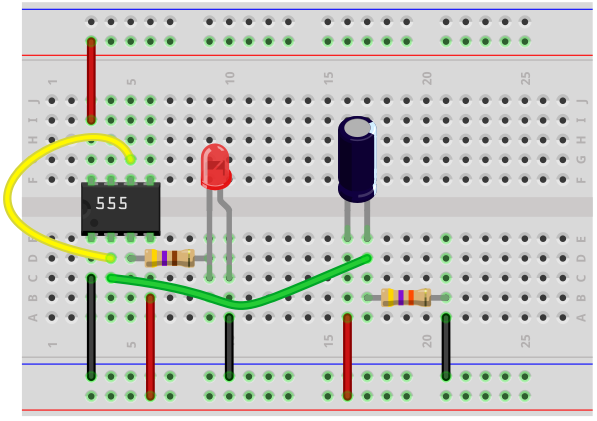﻿ How to Build a Delay Before Turn On Circuit with a 555 Timer ﻿# How to Build a Delay Before Turn On Circuit with a 555 TimerIn this circuit, we will show how to build a delay before turn on circuit with a 555 timer chip.

A delay before turn on circuit is a circuit that once you apply power to it doesn't turn on the output right away. There is a delay before the output turns on. For this circuit, it's a few seconds delay. Once these few seconds pass, then the output turns on.

A delay circuit can be useful for any circuit that needs a delay before output turn on.

How we achieve the delay effect is through the use of a capacitor. Basically the capacitor takes time to charge up. This time is what creates the delay.

### Components Needed

• 555 Timer Chip
• 47KΩ resistor
• 100μF capacitor
• 470Ω resistor
• LED

The 555 timer can be obtained very cheaply from pretty much any electronic retailer.

The 555 timer is an 8-pin chip.

The pinout of the 555 timer is shown below.The 555 timer requires a power supply voltage of 4.5-16V. We connect this voltage to the VCC pin, pin 8, and we connect GND, pin 1, to ground.

The only other pins we use are the trigger pin, the output pin, the reset pin, and the threshold pin.

Pin 2 is the trigger pin. It works like a starter pistol to start the 555 timer running. The trigger is an active low trigger, which means that the timer starts when voltage on pin 2 drops to below 1/3 of the supply voltage. When the 555 is triggered via pin 2, the ouptut on pin 3 goes high.

Pin 3 is the output pin. 555 timer's output is digital in nature. It is either high or low. The output is either low, which is very close to 0V, or high, which is close to the supply voltage that's placed on pin 8. The output pin is where you would connect the load that you want the 555 timer to power. This may be an LED, for instance.

Pin 4 is the reset pin. This pin can be used to restart the 555 timer's timing operation. This is an active low input, just like the trigger input. Thus, pin 4 must be connected to the supply voltage of the 555 timer to operate. If it is momentarily grounded, the 555 timer's operation is interrupted and won't start again until it's triggered again via pin 2.

Pin 6 is the threshold pin. The purpose of this pin is to monitor the voltage across the capacitor that's discharged by pin 7. When this voltage reaches 2/3 of the supply voltage (VCC), the timing cycle ends, and the output on pin 3 goes low.

If you want to know all the pinout of the 555 timer in full depth, what each pin is and what each pin does, see 555 Timer Pinout.

In this circuit, we will connect the 555 timer to produce a delay before the output goes HIGH.

The connections are shown below.

### 555 Timer Delay Before Turn On Circuit

The 555 timer delay before turn on circuit we will build is shown below.The breadboard schematic of the above circuit is shown below.To connect power to the 555 timer chip, we connect about 5-15V to VCC, pin 8 and we connect pin 1 to ground.

We connect a 100μF capacitor to the positive voltage supply and then to pin 2. We connect pin 6 to pin 2 and then connect a 47K resistor to ground.

We connect pin 4, the reset pin, to the VCC. The reset pin is active low. So in order for reset not to be triggered, we connect this pin permanently HIGH.

Pin 3 is the output pin. Therefore, we connect the LED along with the 470Ω resistor.

### How the Circuit Works

How this circuit works is through the RC network. The combination of the resistor and capacitor forms the RC network. This network determines the length of time it takes to charge the capacitor.

The reason why the circuit doesn't turn on automatically is because pin 2, the trigger pin, initially when the power turns on, is HIGH. This is because the capacitor hasn't charged up yet. Until the capacitor charges up, this pin is HIGH. Since the trigger pin is active LOW, the output will be off until this pin goes LOW. As the capacitor charges up and gets near the supply voltage it is connected to, the voltage at pin 2 decreases. When the voltage at pin 2 gets below 1/3 of the supply voltage, the pin is now LOW. When it is LOW, this is when the output goes HIGH and the LED turns on.

There is a delay of about 7 seconds with this circuit. once you turn the power on, the LED doesn't turn on until about after 7 seconds.

The RC network is what determines this. The bigger the resistor and capacitor value, the longer the delay. So if you want to increase the time of the delay, you would choose a bigger resistor and capacitor. If you want to shorten the time of the delay, you would choose a smaller resistor and capacitor value.

And this is how a delay before turn on circuit works with a 555 timer.

To see how this circuit works in real life, see the video below.

Related Resources

How to Build a Delay Before Turn Off Circuit with a 555 Timer